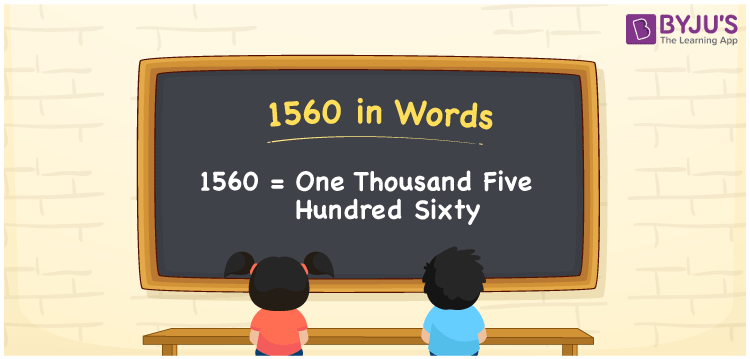# 1560 in Words

1560 in words is written as “One Thousand Five Hundred Sixty”. In Maths, 1560 is a cardinal number that expresses a quantity. If the price of a Travelling bag is Rs.1560, then it can be represented as Rupees One Thousand Five Hundred Sixty, in English. Learn more about Numbers in Words at BYJU’S.

 1560 in Words One Thousand Five Hundred Sixty One Thousand Five Hundred Sixty in Numbers 1560

## 1560 in English Words## How to Write 1560 in Words?

The number 1560 in words can be written using a place value system, where we can identify the position of each digit. Since 1560 is a four-digit number, thus the place value of the digits is given by:

 Thousands Hundreds Tens Ones 1 5 6 0

From the above table,

1 → Thousands place

5 → Hundreds place

6 → Tens

0 → Ones place

Hence, when we read the number from right to left, it is One Thousand Five Hundred Sixty.

### Expanded Form of 1560

We can write the expanded form as:

1 x Thousand + 5 × Hundred + 6 × Ten + 0 × One

= 1 x 1000 + 5 × 100 + 6 × 10 + 0 × 1

= 1000 + 500 + 60 + 0

= One Thousand Five Hundred Sixty

1560 is a whole number that is succeeded by 1559 and preceded by 1561. Learn more about the number 1560 below:

• 1560 in Words – One Thousand Five Hundred Sixty
• Is 1560 an odd number? – No
• Is 1560 an even number? – Yes
• Is 1560 a perfect square number? – No
• Is 1560 a perfect cube number? – No
• Is 1560 a prime number? – No
• Is 1560 a composite number? – Yes

## Frequently Asked Questions (FAQs) on 1560 in words

Q1

### What is 1560 in words?

1560 in words is given by One Thousand Five Hundred Sixty.
Q2

### What is the rule to write 1560 in words?

1560 in words is written based on the place values of each digit. 1 is at thousands, 5 at hundreds, 6 at tens and 0 at ones. Thus, reading from right to left, it is One Thousand Five Hundred Sixty.
Q3

### What is the value of 1560 + 100 in words?

1560 + 100 = 1660, i.e., One thousand six hundred sixty in words.
Q4

### How 1560 is written in words on a cheque?

On a cheque 1560 is written as One Thousand Five Hundred Sixty.
Q5

### Is 1560 an even Number?

Yes.1560 is an even number.